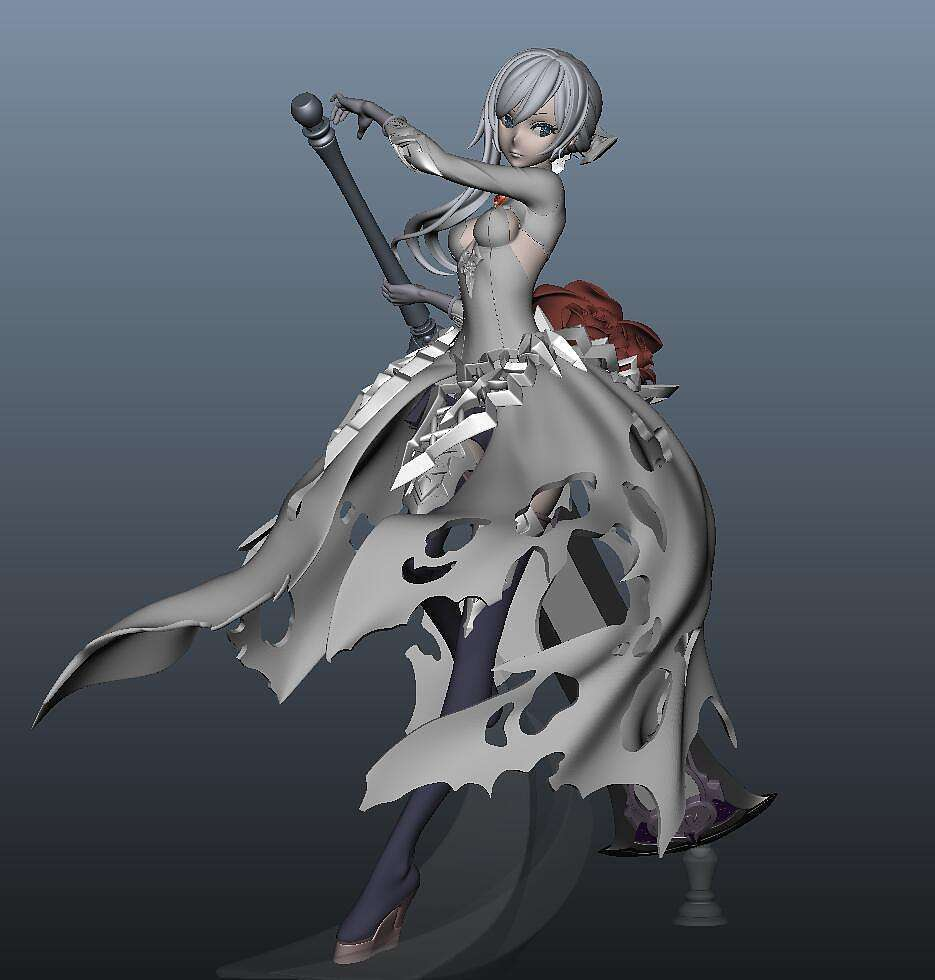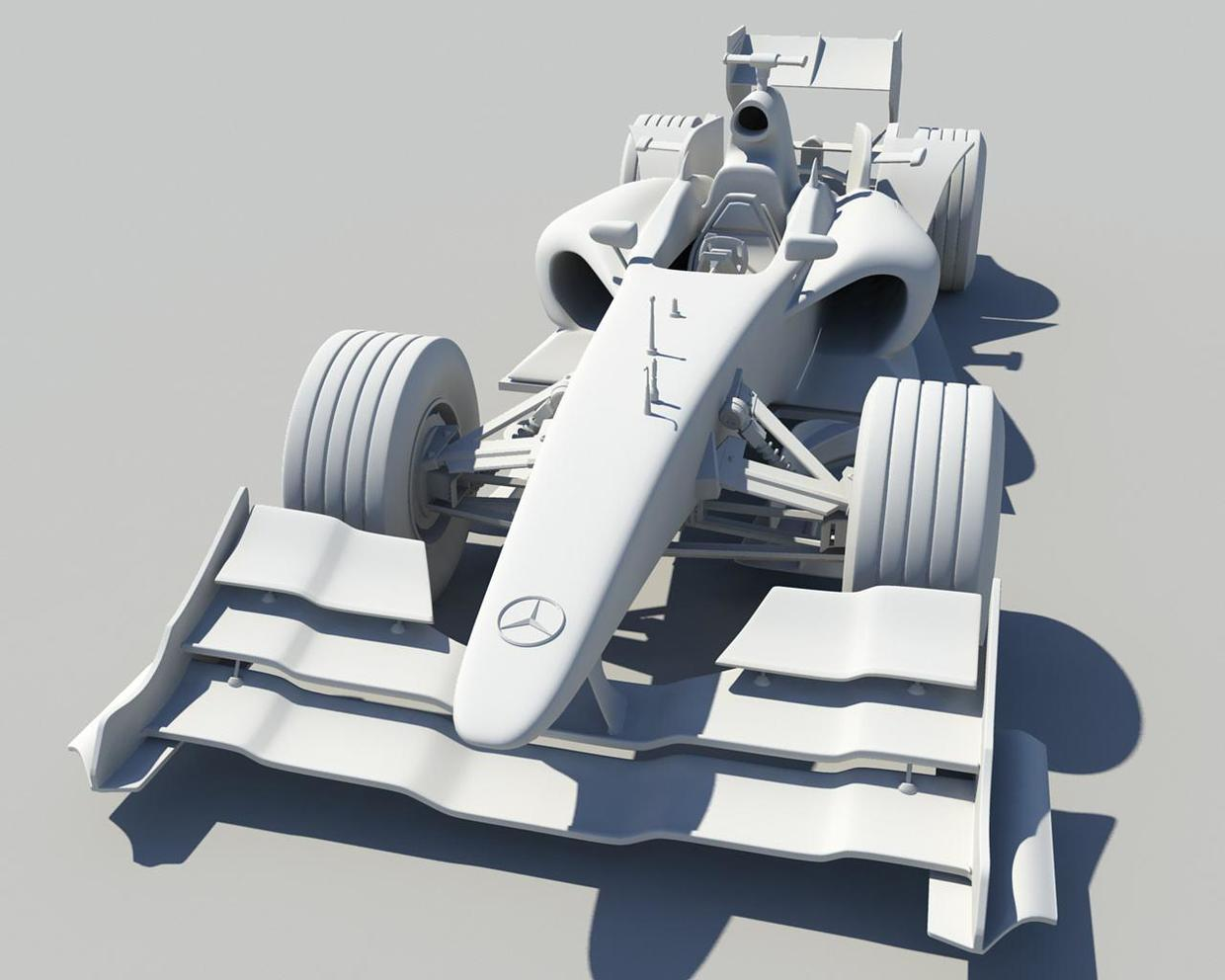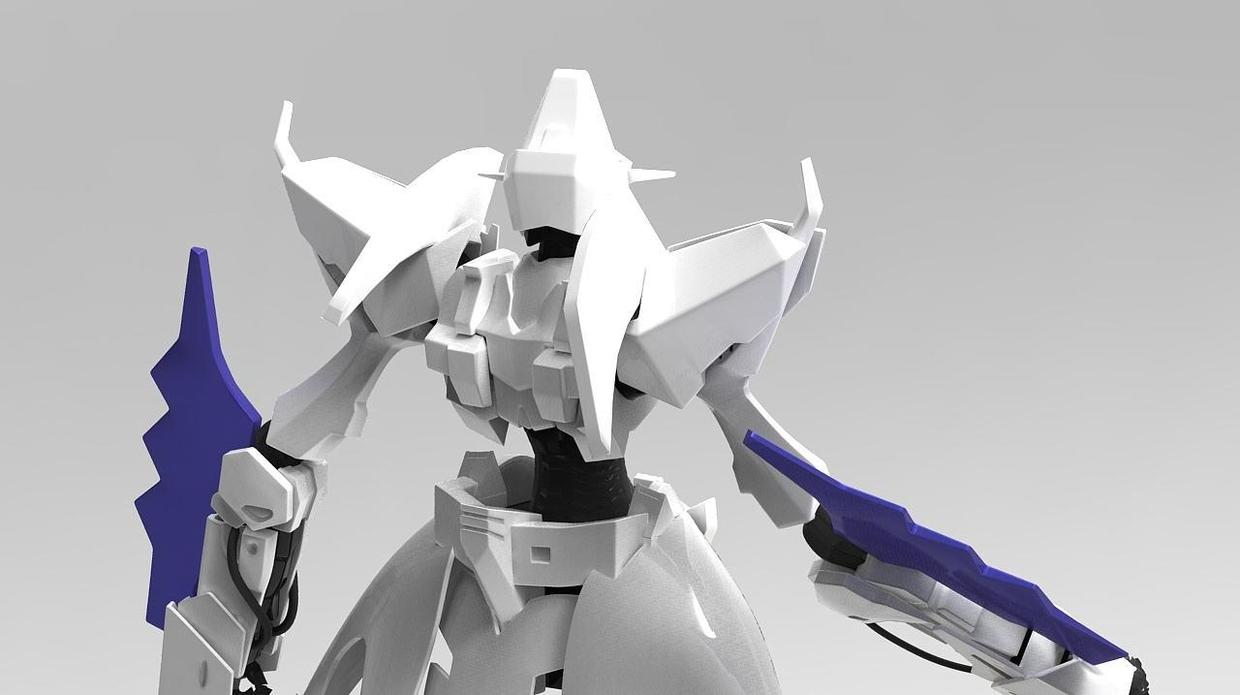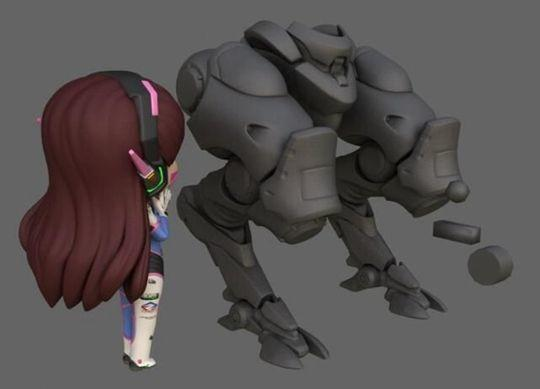• ## 凸，凹多边形定义

千次阅读 2017-04-27 10:29:35
多边形(Polygon)： 由三条以上的直线所组成的形状为多边形。 凸多边形(Convex Polygon):每个内角(Interior Angle)都是锐角(Acute Angle)或钝角(Obtuse Angle),也就是没有大于180°的优角(Reflexive Angle)搜索的...
多边形(Polygon)： 由三条以上的直线所组成的形状为多边形。
凸多边形(Convex Polygon):每个内角(Interior Angle)都是锐角(Acute Angle)或钝角(Obtuse
Angle),也就是没有大于180°的优角(Reflexive Angle)搜索的多边形。
凹多边形(Concave Polygon):至少有一个优角(Reflexive Angle)的多边形。
正多边形：(Regular Polygon)每条边相等，每个角也相等的多边形。
凸多边形，就是把一个多边形任意一边向两方无限延长成为一条直线，如果多边形的其他各边均在此直线的同旁，那么这个多边形就叫做凸多边形，也可以理解为通过凸多边形的任意一条边作平面，并与此多边形所在的平面相异，那么凸多边形的其他所有部分都在所作平面的同一侧。
凹多边形就是把一个各边不自交的多边形任意一边向两方无限延长成为一直线，如果多边形的所有边中只要有一条边向两方无限延长成为一直线时，其他各边不在此直线的同旁，那么这个多边形就叫做凹多边形。
像正方形，是凸变形。 像五角星是凹变形
展开全文• 判断一个多边形是凸多边形还是凹多边形 输入： 输入包含多组测试数据，每组数据占2行，首先一行是一个整数n，表示多边形顶点的个数，然后一行是2×n个整数，表示逆时针顺序的n个顶点的坐标（xi,yi），n为0的...


题目：

判断一个多边形是凸多边形还是凹多边形

输入：

输入包含多组测试数据，每组数据占2行，首先一行是一个整数n，表示多边形顶点的个数，然后一行是2×n个整数，表示逆时针顺序的n个顶点的坐标（xi,yi），n为0的时候结束输入。

输出：

对于每个测试实例，如果地块的形状为凸多边形，请输出“convex”,否则输出”concave”，每个实例的输出占一行。

解析：

向量的数量积（内积，点积）
向量的向量积（外积，叉积) ，符合右手规则。

向量积是一个伪向量，定义其方向垂直于进行叉积的两个向量且满足右手规则。

向量叉积的几何意义

1.向量的叉积的模表示这两个向量围成的平行四边形的面积。

设矢量P = ( x1, y1 )，Q = ( x2, y2 )，

则矢量叉积定义为由(0,0)、p1、p2和p1+p2所组成的平行四边形的带符号的面积，即：P×Q = x1*y2 – x2*y1，其结果是一个伪矢量。

显然有性质 P × Q = – ( Q × P ) 和 P × ( – Q ) = – ( P × Q )。

2.叉积的一个非常重要性质是可以通过它的符号判断两矢量相互之间的顺逆时针关系：

若 P × Q > 0 , 则P在Q的顺时针方向
若 P × Q < 0 , 则P在Q的逆时针方向

若 P × Q = 0 , 则P与Q共线，但可能同向也可能反向

叉积的方向与进行叉积的两个向量都垂直，所以叉积向量即为这两个向量构成平面的法向量。

3.如果向量叉积为零向量，那么这两个向量是平行关系。

4.因为向量叉积是这两个向量平面的法向量，如果两个向量平行无法形成一个平面，其对应也没有平面法向量。所以，两个向量平行时，其向量叉积为零。

代码：

#include<stdio.h>
#include<stdlib.h>

struct xy
{
int x;
int y;
}d;

int g(int a,int b,int c)
{
int t;
//公式:s=(x1-x3)*(y2-y3)-(x2-x3)*(y1-y3)
//当s>0时,p1,p2,p3三个点呈逆时针
//当s<0时,p1,p2,p3三个点呈顺时针
t=(d[a].x-d[c].x)*(d[b].y-d[c].y)-(d[b].x-d[c].x)*(d[a].y-d[c].y);
return t;
}

int main()
{
int i,t,n;
while(scanf("%d",&n),n)
{
for(i=0;i<n;i++)
{
scanf("%d %d",&d[i].x,&d[i].y);
}
for(i=0;i<n;i++)
{
//模n是因为当i=n-1的时候n+1,n+2会超出数据范围,所以从头开始为最后一个点和第一二个点判断直线的走向
t=g(i%n,(i+1)%n,(i+2)%n);
if(t<0)
break;
}
if(t>=0)
printf("convex\n");
else
printf("concave\n");
}
return 0;
}

此文章转载自：https://www.cnblogs.com/wushuaiyi/archive/2013/12/05/3458659.html

---------------------
作者：线上幽灵
来源：CSDN
原文：https://blog.csdn.net/chen134225/article/details/82459467
版权声明：本文为作者原创文章，转载请附上博文链接！

展开全文• ## 多边形Polygon

千次阅读 2017-02-21 22:34:59
多边形 大家都很熟悉，我们把他定义为 多条边首尾连接的封闭图形，关于多边形的算法有很多，比如 是否为凸多边形、直线与多边形求交、填充算法、三角化等，具体的算法原理作者就不再细讲，直接给出作者以前写的具体...
        多边形 大家都很熟悉，我们把他定义为 多条边首尾连接的封闭图形，关于多边形的算法有很多，比如 是否为凸多边形、直线与多边形求交、填充算法、三角化等，具体的算法原理作者就不再细讲，直接给出作者以前写的具体代码，大家作为参考：
封装的Math工具类（MathTool.h），这里也用到了之前文章里的（Tuple2.h和Tuple3.h）

/* Math工具类 - 包含基本数学算法
linolzhang, 2010.3.11
*/

#ifndef __MATH_TOOL_H_INCLUDED__
#define __MATH_TOOL_H_INCLUDED__

#include "Tuple2.h"
#include "Tuple3.h"
#include <vector>

// 点p在线段(pa,pb)的Side  - 左侧（内侧）  1
//                         - 右侧（外侧）  -1
//                         - 线上          0
inline int LineSide(float x,float y,float xa,float ya,float xb,float yb)
{
float f = (xb-xa)*(y-ya) - (x-xa)*(yb-ya);
if(f>0)
return 1;
else if(f<0)
return -1;
else
return 0;
};
inline int LineSide(const Point2D& p,const Point2D& pa,const Point2D& pb)
{
float f = (pb.x-pa.x)*(p.y-pa.y) - (p.x-pa.x)*(pb.y-pa.y);
if(f>0)
return 1;
else if(f<0)
return -1;
else
return 0;
};

// 三维点p在线段(pa,pb)的Side  - 左侧（内侧）  1
//                             - 右侧（外侧）  -1
//                             - 线上          0
inline int LineSide(const Point3D& p,const Point3D& pa,const Point3D& pb,const CVector3& norm)
{
CVector3 edge(pb-pa);  // edge
CVector3 vFace = norm.getCrossProduct(edge);  // 线内方向

CVector3 pointDir(p-pa);
float f = vFace.getDotProduct(pointDir);

if(f>0)
return 1;
else if(f<0)
return -1;
else
return 0;
};

// 查看点是否在多边形区域 - side叉乘判断
inline bool IsPointInConvexPolygon(const Point2D& point,const std::vector<Point2D>& polygon)
{
// 遍历
for(int i=0;i<(int)polygon.size();i++)
{
int nextIndex = i+1>(int)polygon.size()-1 ? 0 : i+1;

//int lineSide = LineSide(point.X,point.Y,polygon[i].X,polygon[i].Y,polygon[nextIndex].X,polygon[nextIndex].Y);
int lineSide = LineSide(point,polygon[i],polygon[nextIndex]);
//int lineSide = LineSide(Point3D(point.x,point.y,0),Point3D(polygon[i].x,polygon[i].y,0),Point3D(polygon[nextIndex].x,polygon[nextIndex].y,0),CVector3(0,0,1));
if( lineSide==-1)
return false;   // 在外侧
}
return true;
};

// 查看点是否在多边形区域
inline bool IsPointInPolygon(const Point2D& point,const std::vector<Point2D>& polygon)
{
size_t i, j;
bool status = false;

for( i=0,j=polygon.size()-1; i<polygon.size(); j=i++ )
{
// 是否在线段的y范围内
bool bInLineY = polygon[i].y<=point.y && point.y<polygon[j].y || polygon[j].y<=point.y && point.y<polygon[i].y;
if(!bInLineY)
continue;

float fSlope = (polygon[j].x - polygon[i].x) / (polygon[j].y - polygon[i].y);  // 以y轴为基准的斜率
float x = (point.y - polygon[i].y) * fSlope + polygon[i].x;

if ( bInLineY && point.x < x )
status = !status;
}
return status;
};
// 点Point到线段(Point1,Point2)的最短距离 - result最近点
inline float DistanceToSegment(const Point2D& point, const Point2D& point1,const Point2D& point2, Point2D& result=Point2D(0.0f,0.0f))
{
CVector2 v1(point.x-point1.x,point.y-point1.y);
CVector2 v2(point.x-point2.x,point.y-point2.y);
CVector2 v12(point2.x-point1.x,point2.y-point1.y);
float k = ( v1.x*v12.x+v1.y*v12.y ) / v12.getLengthSquared();  // |v1|*cosA / |v12|
if( k<0 )       // 钝角，point1为最近点
{
result = point1;
return v1.getLength();
}
else if( k>1 )  // |v1|*cosA>|v12|, 投影长度大于v12长度，point2为最近点
{
result = point2;
return v2.getLength();
}
else            // 垂足在线段上
{
result = point1 + k * v12;
return Point2D(point-result).getLength();
}
}
// 点Point到线段(Point1,Point2)的最短距离 - result最近点
inline float DistanceToSegment(const Point3D& point, const Point3D& point1,const Point3D& point2, Point3D& result=Point3D(0.0f,0.0f,0.0f))
{
CVector3 v1 = point - point1;
CVector3 v2 = point - point2;
CVector3 v12 = point2 - point1;
float k = ( v1.getDotProduct(v12) ) / v12.getLengthSquared();  // |v1|*cosA / |v12|
if( k<0 )       // 钝角，point1为最近点
{
result = point1;
return v1.getLength();
}
else if( k>1 )  // |v1|*cosA>|v12|, 投影长度大于v12长度，point2为最近点
{
result = point2;
return v2.getLength();
}
else            // 垂足在线段上
{
result = point1 + k * v12;
return Point3D(point-result).getLength();
}
}

// -----------------------------------------------------------------------------

// 射线与平面求交 - 根据 射线原点、射线方向向量 p(t)=origin + t * rayDir;
//                       平面法向、平面上一点
//                       求出射线与平面 交点参数 t
inline bool RayIntersectPlane(const Point3D& origin,const CVector3& rayDir,
const CVector3& norm,const Point3D& p0,float& t)
{
// 计算射线与平面法向夹角
float b = norm.getDotProduct(rayDir);
if (fabs(b) < 0.00001f)      // ray is parallel to triangle plane
return false;

// 计算p0到 射线起始点 的向量
CVector3 w0 = origin - p0;
float a = -( norm.getDotProduct(w0) );

// get intersect para of ray with plane
t = a / b;
return true;
}
#endif

Polygon2D封装类（Polygon2D.h），包含 包围盒、面积、到点的距离、三角化等操作。
/* 多边形定义 - 2D
linolzhang, 2010.2.10
*/

#ifndef POLYGON2D_H
#define POLYGON2D_H

#include "Tuple2.h"
#include "MathTool.h"

#include <float.h>
#include <vector>
#include <list>

// 定义多边形包围盒
struct BoundBox2D
{
BoundBox2D() : minX(FLT_MAX),maxX(-FLT_MAX),minY(FLT_MAX),maxY(-FLT_MAX)
{
}
float minX,maxX;
float minY,maxY;
};

// 多边形类，二维表示
class Polygon2D
{
public:
Polygon2D(const std::vector<Point2D>& pointList)
{
m_PointList = pointList;
_calcBoundBox();
}
~Polygon2D()
{
}

// 判断多边形是否为凸多边形 - 假设为逆时针
bool isConvex()
{
// 遍历取相邻三个顶点，LineSide一致说明是凸多边形，否则为凹多边形
int result0 = LineSide(m_PointList,m_PointList,m_PointList);
for(size_t i=1;i<m_PointList.size();i++)
{
int index1 = i;
int index2 = (i+1)%m_PointList.size();
int index3 = (i+2)%m_PointList.size();

// 判断条件：第三个点在前两个点连线的内侧
int result = LineSide(m_PointList[index3],m_PointList[index1],m_PointList[index2]);
if(result!=result0)
return false;
}
return true;
}

// 得到点到多边形的距离
float getDistance(const Point2D& point)
{
float fDistance = FLT_MAX;
for (size_t i=0; i<m_PointList.size(); i++)
{
Point2D point1 = m_PointList[i];
Point2D point2 = m_PointList[ (i+1) % m_PointList.size()];

float fMinDistance = DistanceToSegment(point, point1,point2);
if (fMinDistance < fDistance)
{
fDistance = fMinDistance;
}
}
return fDistance;
}
// 得到中心点
Point2D getCenterPoint()
{
Point2D center(0.0f,0.0f);
for(size_t i=0;i<m_PointList.size();i++)
{
center.x += m_PointList[i].x;
center.y += m_PointList[i].y;
}
center.x /= m_PointList.size();
center.y /= m_PointList.size();

return center;
}
// 得到包围盒
const BoundBox2D& getBoundBox()
{
return m_BoundBox;
}

// 判断点是否在多边形内
bool isPointIn(const Point2D& point)
{
// 无法构成标准区域的情况
if(m_PointList.size()<3)
return false;

// 过滤区域以外的人员 - 先判断包围盒
if( point.x<m_BoundBox.minX || point.x>m_BoundBox.maxX ||
point.y<m_BoundBox.minY || point.y>m_BoundBox.maxY )
return false;

// 判断点是否在平面区域内
if( !IsPointInPolygon(point,m_PointList) )
return false;
return true;
}

{
bool bInPolygon = isPointIn(center);
if(bInPolygon)  // 中心点在多边形内部 - 有交
{
return true;
}
else  // 中点在多边形外部
{
{
return false;
}
else
{
float fDistance = getDistance(center);
return true;

return false;
}
}
}

// 计算多边形面积
float calcArea()
{
// 首先计算三角划分
std::vector<int> trianList;
trianglation(trianList);

// 计算每个三角形面积，然后求和 - 三边长，利用Heron公式
float fArea = 0.0f;
for(size_t i=0;i<trianList.size();i+=3)
{
float a = Distance(m_PointList[trianList[i]],m_PointList[trianList[i+1]]);
float b = Distance(m_PointList[trianList[i+1]],m_PointList[trianList[i+2]]);
float c = Distance(m_PointList[trianList[i]],m_PointList[trianList[i+2]]);

float s = 0.5f * (a+b+c);
fArea += sqrtf( s*(s-a)*(s-b)*(s-c) );
}
return fArea;
}

// 三角化 - trianList记录三角形顶点索引（0,1,2; 3,4,5; ……）
void trianglation(std::vector<int>& trianList)
{
bool bIsConvex = isConvex();  // 是否为凸多边形

// 首先遍历顶点，加入标记链表
std::vector<int> flagList;
for(size_t i=0;i<m_PointList.size();i++)
{
flagList.push_back(i);  // 记录顶点索引
}

// 循环处理顶点 - 直到顶点个数处理完成
while(true)
{
// 结束条件
if(flagList.size()==3)
{
trianList.push_back(flagList);
trianList.push_back(flagList);
trianList.push_back(flagList);
break;
}

// 遍历取相邻三个顶点，找到能构成三角形的合适的顶点
for(size_t i=0;i<flagList.size();i++)
{
size_t i1= (i+1)%flagList.size();
size_t i2 = (i+2)%flagList.size();
int index1 = flagList[i];
int index2 = flagList[i1];
int index3 = flagList[i2];

// 1.判断是否是凸点 - 判断条件：第三个点在前两个点连线的内侧
int result = LineSide(m_PointList[index3],m_PointList[index1],m_PointList[index2]);
if(result!=1)  // 逆时针 1为左侧
continue;

// 2.判断是否有没有其它顶点在生成的三角形中 - 凸多边形不需要计算
if(!bIsConvex)
{
bool bIncludeOtherVertex = false;
for(size_t j=0;j<flagList.size();j++)
{
if(j==i||j==i1||j==i2)  // 过滤掉当前点
continue;

int pointIndex = flagList[j];
if( LineSide(m_PointList[pointIndex], m_PointList[index1],m_PointList[index2])==1 &&
LineSide(m_PointList[pointIndex], m_PointList[index2],m_PointList[index3])==1 &&
LineSide(m_PointList[pointIndex], m_PointList[index3],m_PointList[index1])==1 )
{
bIncludeOtherVertex = true;
break;
}
}
if(bIncludeOtherVertex)  // 构成的三角形包含其它顶点，不符合要求，继续查找
continue;
}

// 处理三角形剖分
flagList.erase( flagList.begin() + i1 );  // 删除中间索引记录
trianList.push_back(index1);
trianList.push_back(index2);
trianList.push_back(index3);
break;
}

}

// 三角化完成
}

protected:
// 计算包围盒
void _calcBoundBox()
{
// 遍历边列表
for(size_t i=0;i<m_PointList.size();i++)
{
if( m_PointList[i].x < m_BoundBox.minX )
m_BoundBox.minX = m_PointList[i].x;
if( m_PointList[i].x > m_BoundBox.maxX )
m_BoundBox.maxX = m_PointList[i].x;

if( m_PointList[i].y < m_BoundBox.minY )
m_BoundBox.minY = m_PointList[i].y;
if( m_PointList[i].y > m_BoundBox.maxY )
m_BoundBox.maxY = m_PointList[i].y;
}
}

public:
std::vector<Point2D> m_PointList;    // 点列表
BoundBox2D m_BoundBox;               // 包围盒
};

#endif


展开全文凸包 Polygon
• 多边形网格定义每个3D角色和对象的形状和轮廓，无论是用于3D动画电影，广告还是视频游戏。 这个解释有点难以理解，但是多边形网格背后的几何结构很容易理解。大型模型是由较小的相互连接的平面（通常是三角形或矩形...
在计算机图形学中，多边形网格是构成3D对象的顶点，边缘和面的集合。多边形网格定义每个3D角色和对象的形状和轮廓，无论是用于3D动画电影，广告还是视频游戏。
这个解释有点难以理解，但是多边形网格背后的几何结构很容易理解。大型模型是由较小的相互连接的平面（通常是三角形或矩形）构建而成的，它们像3D拼图一样契合在一起。多边形网格中的每个顶点都存储x，y和z坐标信息。然后，该多边形的每个面都包含表面信息，渲染引擎将这些表面信息用于计算闪电和阴影（以及其他信息）。
多边形网格可以用于几乎所有对象的建模。而且还可以实时生成多边形网格，使其既完美又不失真实。因此，它们在计算机图形世界中被广泛使用。深入探讨多边形网格
对多边形网格进行建模的想法是用线和多边形对任何物体的3D表面进行重现。然后，艺术家可以通过增加网格中的顶点数量来随意添加尽可能多的细节。这方面的唯一限制大概就是电脑的性能罢。
在3D世界中，有几个创建多边形网格的程序。最受欢迎的程序是Blender，Maya和3ds Max。这些都为3D网格的建模，纹理化，装配和动画化提供了工具。这些都可以根据项目进行定制以用于视频游戏或电影。尽管大多数3D对象是实体，但不一定必须是多边形网格。单个平面可用于创建“薄”网格。为了进行优化，大多数网格被渲染为多边形四边形（四个相连的顶点），这些多边形被计算机分割成三角形。
任何多边形网格的每个面都具有两个侧面：正面和背面。正面用于计算网格的表面角度，而背面则意味着对摄像机隐藏。如果出了点问题并且相机看到背面，则将无法正确渲染。这可能是您在经典PlayStation或N64游戏中看到的一些旧故障块的原因。
网格还包含有关其UV坐标的数据。这些用于在网格表面上正确显示纹理。通过UV展开网格（可以使框变平或展开），美术师可以在表面上绘制纹理和颜色，然后重新形成3D形状。多边形网格确实有其局限性。用一系列直线逼近曲面非常困难。有机形状需要大量的顶点。而例如头发和液体之类的物体则很难使用多边形网格进行模拟。暗中观察
我们所有喜欢的视频游戏和3D卡通人物都是通过网格制成的。为了创造他们，可以将这些网格“变形”以使其移动，扭曲或旋转。通过这种方式，可以使角色在屏幕上飞来飞去。
在大多数工作室中，一位艺术家会制作一个网格并将其传递给另一位艺术家进行装配和动画处理。每个艺术家都专注于3D动画制作流程的不同部分，但是他们都使用相同的资源。为了创建网格，大多数艺术家将从一幅画开始，该画从多个角度显示他们想要制作的对象。通常，他们至少会看到正面和侧面。通过在建模时在这些视图之间切换，美术师可以更好地创建3d表单，并且准确性更高。
通常最好从对整个对象的低多边形版本建模开始。这意味着减少细节以近似最通用的形式。该模型的低多边形版本可在以后用于创建各种高分辨率版本。对于缺乏处理能力的视频游戏开发者，拥有不同版本的模型非常有用。为了节省内存，大多数工作室都在制作游戏时只在相机关闭时显示高多边形模型。当对象距离很远时，将替换低多边形版本。没有纹理，多边形网格将看起来不多。添加一些颜色可以使其看起来像无聊的雕像，但它仍然只是基本的网格物体。通过纹理，艺术家可以更改模型的外观，使其看起来像各种表面，包括污垢，皮肤或蓝色牛仔裤。
通过使用UV坐标，纹理可以应用于模型的表面。通过仔细地将网格的位置映射到图像上，美术师还可以为每个平面赋予独特的纹理。该UV贴图可以带入任何图像处理程序，并可以编辑为项目所需的任何细节。在某些程序中也可以组合不同的纹理。例如，通过将颜色贴图与镜面贴图叠加，可以显示表面细节的外观。
在许多方面，现代CG世界都是使用多边形网格构建的。如果曾经看过3D动画的话，那么这些应该都不难理解。想自己设计更好看的模型或者对游戏模型的学习有兴趣的，可以关注订阅号：沉迷游戏和建模的灰灰，免费的模型相关学习资料分享给你，快来白嫖吧！


展开全文java python 机器学习 游戏开发
• 退化多边形是多边形的一种，是一种退化的多边形，是指一个多边形在满足多边形定义的临界。退化三角形是指面积为零的三角形。满足下列条件之一的三角形即可称为退化三角形：三个内角的度数为（180°,0°,0°）或（90...
• PTA一道题，依旧没有在规定时间做出来。.../*定义多边形类*/ class polygon { protected: int number;//边数，最多不超过100条边 private: int side_length;//边长数组 public: polygon(); polygon...c++
• 星形多边形什么呢？其实就是在多边形内部存在一个点A，这个点与多边形内任意一点的连线都在多边形内部，就叫做星形多边形，这一个点A就叫做多边形的内核。 我是在看计算几何这本书的时候发现的，当时不是很懂书上...
• 给定一组点的数组，当一个多边形按顺序连接时，发现这个多边形是凸多边形(凸多边形定义)。 思路：我们可以通过判断三角形是顺时针还是逆时针来求解； 具体判断三角形是顺时针还是逆时针参考此篇博文《三角形顺时针...
• 多边形网格定义每个3D角色和对象的形状和轮廓，无论是用于3D动画电影，广告还是视频游戏。 这个解释有点难以理解，但是多边形网格背后的几何结构很容易理解。大型模型是由较小的相互连接的平面（通常是三角形或矩形...3d zbrush
• 该子集定义了包括上述所有点的凸多边形。 该函数考虑对齐或相同的点。 下面是如何使用具有 100 个随机 2D 点的函数的示例。 初始点为蓝色，凸多边形点以红色链接。 ****************** 清除; nouv_list_points3D=...matlab
• ## 多边形切割

千次阅读 2018-06-20 14:30:50
之前基本上遇到的多边形切割问题都是凸多边形问题，而针对凹多边形的切割问题却很少。偶然发现一个做得特别棒的滑动切割的游戏，游戏中主要是使用多边形切割以及多边形碰撞算法。针对多边形切割的实现做了一下研究，...
• ## 多边形扩展算法

千次阅读 2017-06-20 16:35:15扩展 算法
• （Convex Polygon）可以有以下三种定义： 1、没有任何一个内角是优角（Reflexive Angle）的多边形。 2、如果把一个多边形的所有边中，有一条边向两方无限延长成为一直线时，其他 3、凸多边形是一个内部为凸集的简单...
• 多边形极点，定义为，多边形内距离边界最远的点。我这里用来这个点位来标注凹多边形的名称，如下图，计算多边形质心位置时，不一定会落在多边形为内部，此时可以用极点来表示改多边形内部的点。 第三方库, mapbox...
•计算机图形学
• 多边形重心问题 时间限制：3000 ms | 内存限制：65535 KB 难度：5 ...描述 在某个多边形上，取n个点，这n个点顺序给出，按照给出顺序将相邻...如果是一条线段,我们定义面积为0，重心坐标为（0,0）.现在求给出的点
• 多边形游戏是一个单人玩的游戏，开始时有一个由n个顶点构成的多边形。每个顶点被赋予一个整数值， 每条边被赋予一个运算符“+”或“*”。所有边依次用整数从1到n编号。
• 我改进了计算图形直接定义多边形计算程序，请试用，请将试用的评价贴出来。
• //其实不得不说这个问题...在某个多边形上，取n个点，这n个点顺序给出，按照给出顺序将相邻的点用直线连接， （第一个和最后一个连接），所有线段不和其他线段相交，但是可以重合，可得到一个多边形或一条线段或一个多南阳理工OJ
• 该程序生成具有随机边数和随机 (x,y) 坐标的多边形。 如果它们包含在开头定义的“测试”多边形内，它们将保留在屏幕上。 该形状最终将用多边形填充。Java
• 多边形地图 转化地理角色，点ID必须在维度列，双击经纬度，取消聚合度 标记-多边形，地市-颜色，顺序（点ID）-路径，添加注释 ...定义位置 导入自定义地理编码csv文件，格式必定要正确，可以添加层级 ...
• const Point=require('./Point') const Line=require('./Line') //多边形类 function Polygon(a) { if(this instanceof Polygon){ this.n=a.length; this.a=a; }else{ if(a.e...
• 你可以假定给定的点形成的多边形均为简单多边形（简单多边形定义）。换句话说，保证每个顶点处恰好是两条边的汇合点，并且这些边 互不相交 。 示例 1： [[0,0],[0,1],[1,1],[1,0]] 输出： True 示例 2： [[0,0],...
• ## 多边形构建三角形

千次阅读 2019-03-01 13:41:33
本篇文章将介绍怎样将一个多边形剖分成三角形，写这篇博客的背景是由于我想要利用OpenGL ES绘制面，但是OpenGL ES没有给出由多边形构建面的功能，因此为了绘制面，必须将多边形划分成三角形，以下是过程，由于查询的...
• Main package exp03.main; import exp03.shape.RegularPolygon; import java.util.Scanner; public class Main { ... public static void main(String[] ... System.out.println("输入需要创建的多边形对象的个数n:");
• 1、定义：把多边形的顶点表示转化为点阵表示（就是已知多边形的边界，如何找到多边形内部的点，即把多边形内部填上颜色） 2、表示方法：顶点表示和点阵表示 3.顶点表示:是用多边形的顶点序列来表示多边形。 ...
• ## mfc 多边形绘制

千次阅读 2019-05-19 20:23:17
1.头文件定义： //多边形的顶点，使用vector //它能够像容器一样存放各种类型的对象，简单地说，vector是一个能够存放任意类型的动态数组 struct node { //由于都是一起进栈的，所以xs和ys都是对应的 vector<......